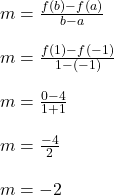## Please Help? For the function f(x) = 2|x – 1|, what is the average rate of change over the interval –1 ≤ x ≤ 1? average rate of change

Question

Please Help? For the function f(x) = 2|x – 1|, what is the average rate of change over the interval –1 ≤ x ≤ 1?
average rate of change:__

in progress 0
4 months 2021-09-05T04:46:21+00:00 1 Answers 2 views 0

## Answers ( )

1. ### Answer:   -2

========================================================

Explanation:

Plug in x = -1 to find that

f(x) = 2|x-1|

f(-1) = 2|-1-1|

f(-1) = 2|-2|

f(-1) = 2*(2)

f(-1) = 4

If you repeat for x = 1, you should find that f(1) = 0

——–

Now use the average rate of change formula below. Effectively, we’re using the slope formula more or less.The average rate of change on this interval is -2

This is the same as finding the slope through the points (-1, 4) and (1, 0).